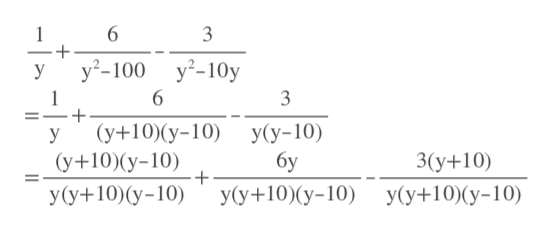# (1/y)+(6/y^2−100)−(3/y^2−10y) how do i find the LCD then solve?

Question
1 views

(1/y)+(6/y^2−100)−(3/y^2−10y)

how do i find the LCD then solve?

check_circle

Step 1

First we factor y^2-100= y^2-10^2= (y+10)(y-10) [used a^2 - b^2=(a+b)(a-b) formula]

Step 2

Then we factor y^2-10y using GCF method.

y^2-10y=y(y-10)

Step 3

LCD= y(y+10)(y-10)

We make ever...help_outlineImage Transcriptionclose6 3 1 -+ у'-100 у?-10у у 6 1 -+ 3 (у+10)(у-10) У(у-10) бу у (у+10)(у-10) 3(у+10) -+ Уу+10)(у-10) У(у+10)(у-10) У(у+10)(у-10) fullscreen

### Want to see the full answer?

See Solution

#### Want to see this answer and more?

Solutions are written by subject experts who are available 24/7. Questions are typically answered within 1 hour.*

See Solution
*Response times may vary by subject and question.
Tagged in

### Other